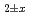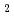# Oxygen potential, oxygen diffusion, and defect equilibria in UOSince the oxygen potential and the oxygen coefficient of UOhave a significant impact on fuel performance, many experimental data have been obtained. However, experimental data of the oxygen potential and the oxygen diffusion coefficient in the high temperature region above 1673 K are very limited. In the present study, we aimed to obtain these data and analyze them by defect chemistry. The oxygen potentials and the oxygen chemical diffusion coefficient of UOwere measured by the gas equilibrium method in the near stoichiometric region at temperatures ranging from 1673 to 1873 K. A data set of oxygen potentials was made together with literature data and analyzed by defect chemistry. The oxygen potential of UOwas determined as a function of O/U ratio and temperature, and an equation representing the relationship was derived. The oxygen chemical diffusion coefficient values obtained in this study were reasonably close to the literature values. The oxygen partial pressure dependence of the oxygen chemical diffusion coefficients was predicted from the evaluated results of the oxygen potential data, but no clear dependence was observed.